# FORM FOUR MATHEMATICS NOTES TOPIC 8: LINEAR PROGRAMMING

Posted on

### FORM FOUR MATHEMATICS STUDY NOTES  TOPIC 8: LINEAR PROGRAMMING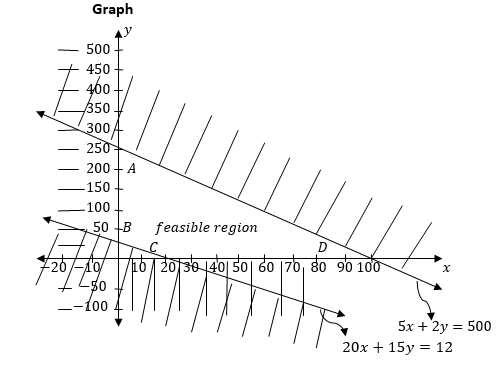Simultaneous Equations
Simultaneous Equation from Word Problems
Form simultaneous equation from word problems
LINEAR PROGRAMMING.
Linear programming – is a branch of mathematics which deals with either minimizing the cost or maximizing the profit.
• It gives the best way of utilizing the scarce resources available.
• It is so called because it only involves equations and inequalities which are linear.
Simultaneous Equation.
One of the methods used in solving linear simultaneous equations is a graphical method. Two linear simultaneous equations in two unknowns can be graphically solved by passing through the following procedures.
1. Draw the two lines which represent the two equations on the xy – plane this is done by deter mining at least two points through which each line passes, the intercept are commonly used
2. Determine the point of intersection of the two lines. This point of intersection is the solution to the system of equations.
FACT:
• If two straight lines are not parallel then they meet at only one point:
• In case the lines do not meet, there is no solution to the corresponding system of simultaneous equations.
Example 1
Graphically solve the following system of simultaneous equations.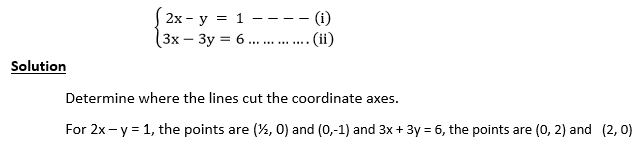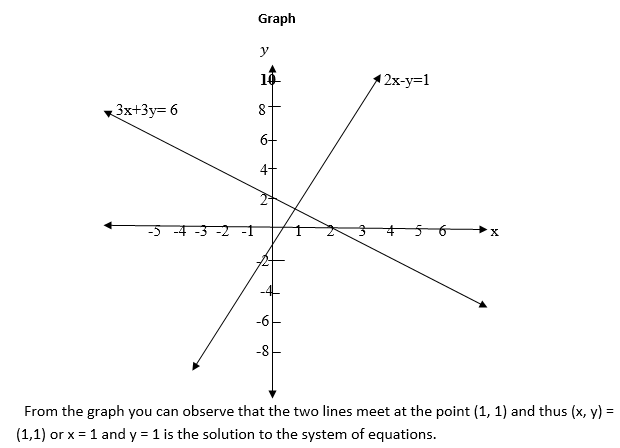Example 2
Find the solution to the following system of simultaneous equations by graphical method.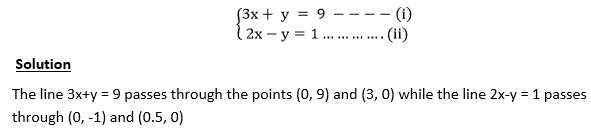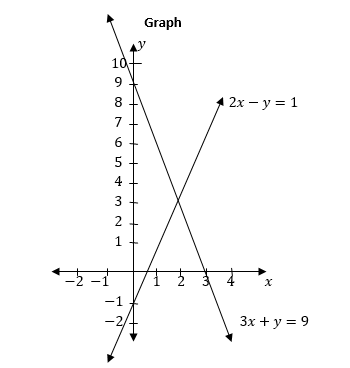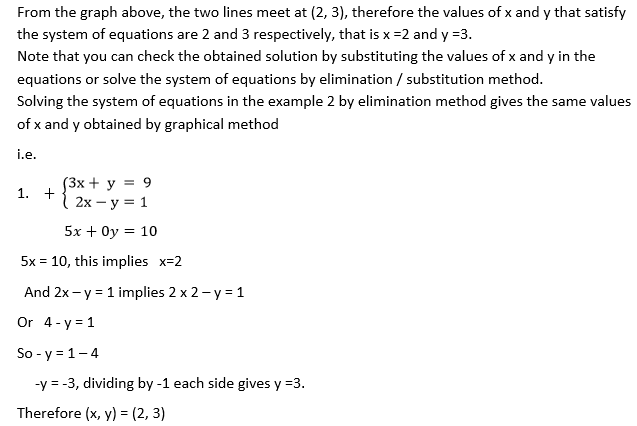Solving Simultaneous Equations Graphically
Solve simultaneous equations graphically
Example 3
Solve the following simultaneous equations graphically and check your solution by a non-graphical method: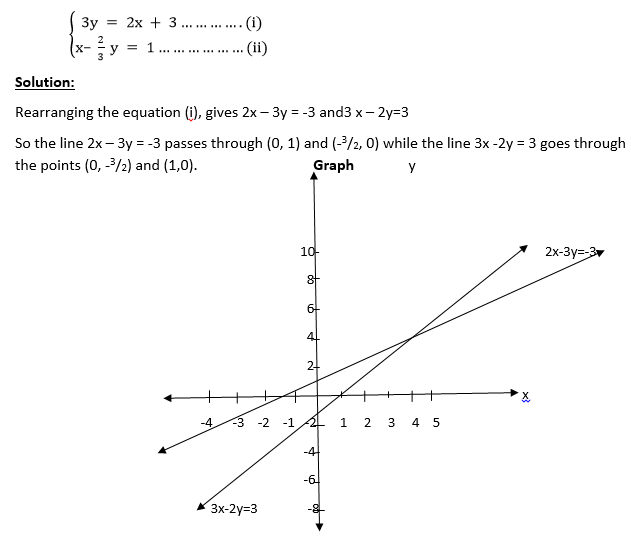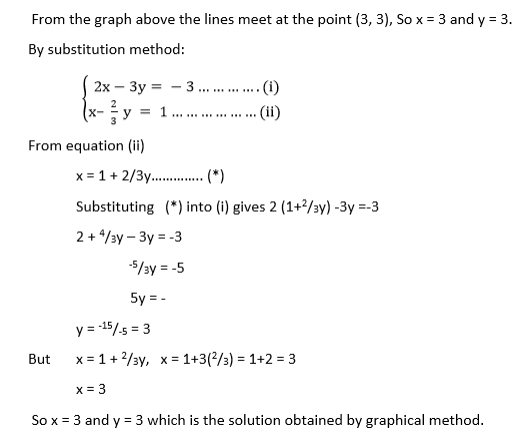Example 4
Find the solution to the following system of simultaneous equations by graphical method.Exercise 1
Find the solution to the following systems of simultaneous equations graphically.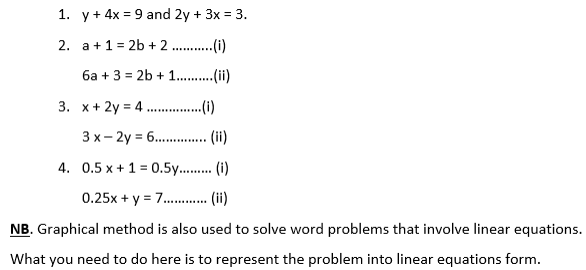Try: Ali paid 34 shillings for 10 oranges and 35 mangoes. Moshi went to the same market and paid 24 shillings for 16 oranges and 18 mangoes. What was the price for a mango and for an orange?